# Why Excel VLOOKUP Not Working – Solved With Examples

Want to know why your Excel VLOOKUP is not working? You are in the right place. This tutorial will help you learn why you are getting VLOOKUP errors in Excel 2019, 2016, 2013, 2010 and 2007.

## Introduction to Excel VLOOKUP Not Working

Most of the Excel gods consider VLOOKUP as their favorite Excel function. If you manage to master this function, then you are qualified to brag a little about your Excel knowhow since you can solve many problems with it.

You can do so much cool stuff if you get to understand the nuts and bolts about the VLOOKUP function, how it works, why it fails to work sometimes, and how to fix any “Excel VLOOKUP not working” problem.

We all know how powerful the VLOOKUP function is, yet it isn’t flawless. Just like most useful things, it too has its side effects. In this topic, I want to talk about some of the things that trip up the VLOOKUP function.

But first, if you are not familiar with this function, you should get to know what it means in plain English. Just click here to read a blow-by-blow guide to the VLOOKUP function.

Now, using the following VLOOKUP cases, let’s see how to deal with the most common VLOOKUP error message: #N/A Error.

## What is #N/A Error in VLOOKUP?

In Excel, #N/A means The Value isn’t Available. This error is very common in performing lookup or statistical functions.

Let’s take the VLOOKUP function, for example, if you use it to look up a range and it can’t find what it is supposed to look for, it will give you the #N/A error message.

Therefore, VLOOKUP #N/A error is Excel’s way of telling you that the lookup value is not found in the first column of the lookup table.

Consider the following VLOOKUP example.

At the right-hand side is a table containing employee information including employee name, occupation, hire date and salary details. At the left-hand side is also a small table that uses the VLOOKUP function to fetch the annual salary based on the name selected.

See screenshot: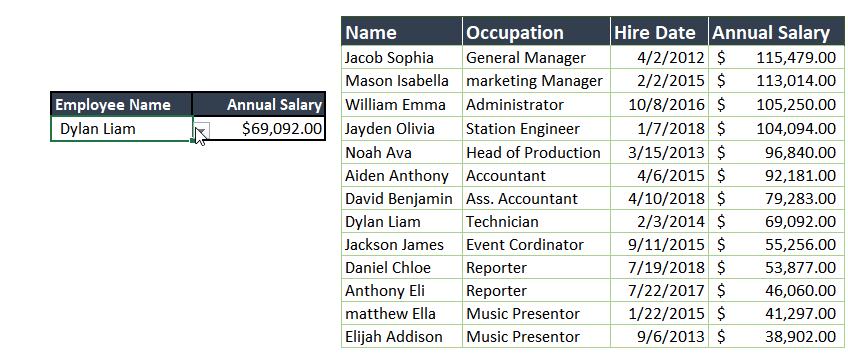## Causes of Excel VLOOKUP Not Working and Solutions

Now let’s look at the circumstances under which the VLOOKUP function will generate an error and how to address these errors.

### #1. You mistyped the lookup value

The lookup value is what the function is supposed to search for in the lookup table.

If for any reason you mistyped the lookup value, the function will not find what you typed and will definitely give you an error message.

For instance, instead of typing Daniel Chloe you mistyped Daniel Kloe as the lookup value. For you, these two names may sound the same but Excel sees them as different names.

See screenshot:As seen in the above screenshot, the VLOOKUP function displayed the #N/A error message when the employee name was mistyped. But when the name was typed correctly, the formula was now able to find the specified employee’s salary.

The solution to this kind of Excel VLOOKUP not working problem is simply to retype the mistyped value correctly.

### #2. Leading and trailing spaces

Like in the above case, the problem disappeared as soon as the user fixe the problem by typing the employee name correctly. Sometimes it is not that easy.

See the example below: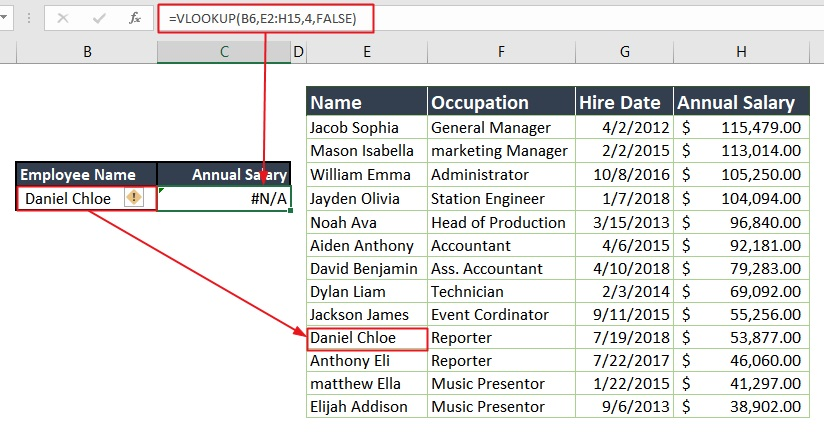Look at the above example. The data, as well as the VLOOKUP formula, is the same as the previous example. Everything looks correct now. This time around the name is spelled correctly. But why do you have this error message? Why can you see this but Excel cannot?

Well, this kind of error takes more than just the eyes to see. This is caused by extra spaces which your eyes can hardly see, especially when dealing with a large volume of data.

Solution:

The solution to this kind of Excel VLOOKUP not working problem is to check and see where there are extra spaces and get rid of them. Extra spaces in the main table (lookup column) can cause the error just like extra spaces in the lookup value.Another way to solve this problem is to use the TRIM function within the VLOOKUP formula.

The trim function removes extra spaces from your cells. Thus, if the extra spaces are in the lookup value (cell B6), instead of looking up B6, look up TRIM(B6).

With this trick, even if there happen to be leading and trailing spaces in your lookup value, the trim function will always handle that for you.However, the extra spaces could be in your lookup table as well. If that’s the case, you’ll make sure you get rid of that too or the error won’t leave you alone.

See screenshot:### #3. Numbers formatted as text

This is another thing to look at when your Excel VLOOKUP formula is not working.

When your function is supposed to search for numbers, but instead it searches for text (or vice versa), Excel will give you an error message.

This kind of errors normally occurs when the data is imported from an external database or maybe you’ve typed an apostrophe before a number.

Numbers can be formatted as text though, but Excel will still alert you with a warning message (sign) that say’s “hey buddy, these are numbers but they are stored as text”.

See screenshot: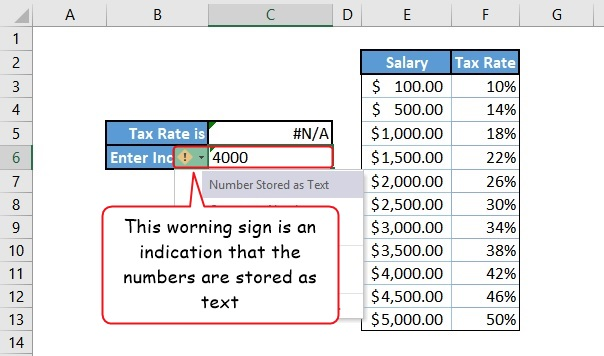Solution:

To solve this problem, all you have to do is format the cell or cells using the number format. If it is just one cell, simply click the warning sign, and select Convert to Number.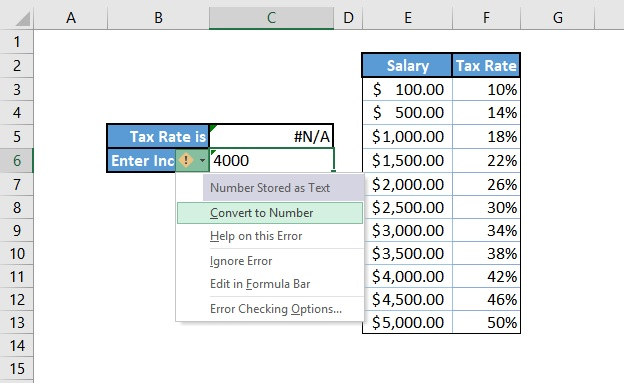But for multiple cells, select them all, and go to the Home tab, in the Number category there’s a drop-down arrow, click it and select the Number format.

### #4. You are using an Approximate Match

In VLOOKKUP, the fourth argument is always optional and you can decide to give it no argument.

You should beware though, as Excel will assign an argument for you by default which in most cases leads to Excel VLOOKUP not working.

Unless you know what you are up to, you should always use FALSE (which means Exact Match) as your fourth VLOOKUP argument.

If you don’t use an Exact Match (i.e. FALSE), then you are heading for trouble.

Why is that?

If you don’t specify the fourth argument (using FALSE for Exact Match), Excel will assume you want an approximate match (using TRUE as a fourth argument).

The approximate match is rarely used when writing real-world VLOOKUP functions. VLOOKUP with an approximate match works completely different from Exact Match which is why you should always stick to using the Exact match.

However, there are some few exceptions. You can use 0 (the number zero) which technically is the same as FALSE. But never leave this argument when writing your VLOOKUP formula because Excel will assign it for you with an approximate match (i.e. True).

Another exception is those who use the VLOOKUP function as a replacement to nested IF statements to solve more sophisticated solutions. Accountants are living examples of those who use VLOOKUP with an approximate match, but that too only 0.99% of the time.

Therefore, if you are not a very clever Excel Rockstar, and you are not also creating a complex system which requires the use of Approximate match, always write your VLOOKUP formula with the Exact match.

### #5. Using an Exact Match

As I mentioned previously, it is always a good practice to write your VLOOKUP function using an exact match as your last argument. But it also means that when Excel doesn’t find the value you are looking for in the lookup table, you will definitely get an error.

The reasons why Excel may not find your search has already been addressed above, which includes mistyping of lookup values, leading and trailing spaces and sometimes when numbers are formatted as text and vice versa.

Now let’s look at the some other VLOOKUP errors that may occur when writing formulas.

## #NAME? error in VLOOKUP

This error is normally caused by a typo. It simply means that Excel can’t find the name of the function you used.Below are some of the circumstances that may lead to #NAME? error in VLOOKKUP.

• When VLOOKUP contains text not enclosed in quotation marks.
• When VLOOKUP function name is misspelled.
• When VLOOKUP formula contains an undefined range or cell name.
• When the VLOOKUP formula omits the colon between the cell addresses of a range reference (lookup table)

When you encounter the #NAME? error in your VLOOKUP formula, you can quickly find a solution by checking the above causes.

## #VALUE! error in VLOOKKUP

This is normally caused by a wrong datatype. Take the lookup value for instance, it only expects a single value, but using a whole range will result in this error. I.e. instead of using cell B3 as the lookup value, you used a cells B3:B5.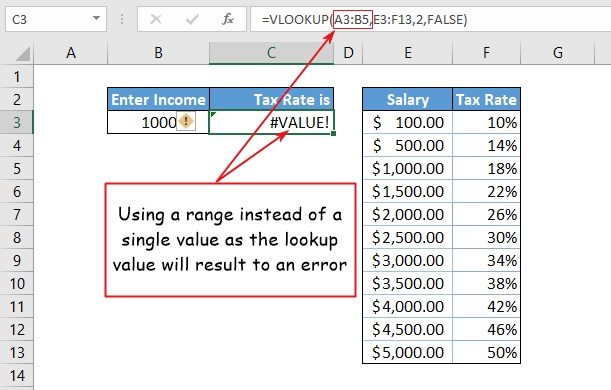## VLOOKUP error handling

You can solve almost every VLOOKUP not working problem if you follow the techniques in this article.

However, you can also fine-tune your formula to make lookup errors cleaner and easier to understand. One good solution is to use the IFERROR function.

Here’s how you can use the IFERROR function to display a message if VLOOKUP fails for any reason:

=IFERROR(VLOOKUP(B3,E3:F13,2,FALSE),”Not Available”)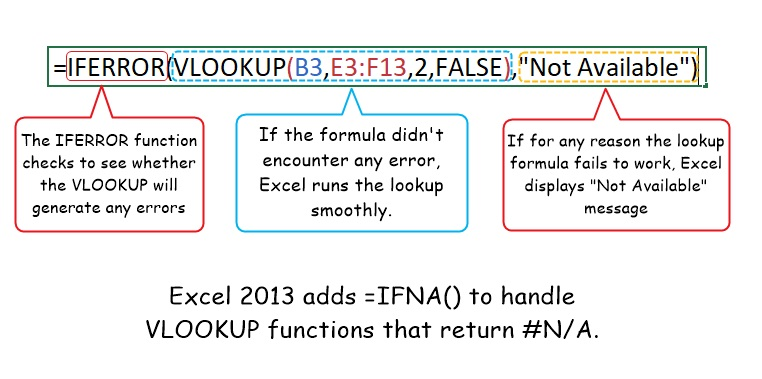What is happening here is very simple. The IFERROR() function checks for any errors in the lookup formula. If there isn’t any error, then Excel runs the lookup. But if there is an error, excel display’s “Not Available” instead of any of the error messages (#N/A, #VALUE! and #NAME?).

Excel 2013 adds =IFNA() function to handle VLOOKUP formulas that return #N/A. The =IFNA() function works the same way as the IFERROR() error. So if you want to catch only #N/A errors, use the =IFNA() instead.